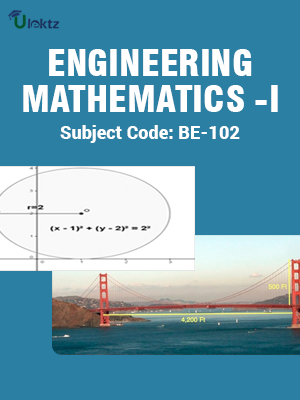•My WalletMy Order
•My Profile
•My Connections
•My Books
•My Videos
•My Tests
•My Calender
•My Messages
•My Shopping Cart
•My Orders
•Account Settings
•Help

# Book Details# ENGINEERING MATHEMATICS-I

 Course Code : BE102 Author : uLektz University : Rajiv Gandhi Proudyogiki Vishwavidyalaya, Madhya Pradesh (RGPV) Regulation : 2010 Categories : Engineering Mathematics Format :ePUB3 (DRM Protected) Type : eBook

FREE

Description :ENGINEERING MATHEMATICS-I of BE102 covers the latest syllabus prescribed by Rajiv Gandhi Proudyogiki Vishwavidyalaya, Madhya Pradesh (RGPV) for regulation 2010. Author: uLektz, Published by uLektz Learning Solutions Private Limited.

Note : No printed book. Only ebook. Access eBook using uLektz apps for Android, iOS and Windows Desktop PC.

##### Topics
###### UNIT I DIFFERENTIAL CALCULUS :

1.1 Expansion of functions by Maclaurin’s and Taylor’s theorem.

1.2 Partial differentiation,Euler’s theorem and its application in approximation and errors,Maxima and Minima of function of two variables

1.3 Curvature : Radius of curvature, centre of curvature.

###### UNIT II INTEGRAL CALCULUS :

2.1 Definite Integrals : Definite Integrals as a limit of a sum ,Its application in Summation of series, Beta and Gamma Functions

2.2 Double and Triple Integrals

2.3 Change of Order of Integration

2.4 Area,Volume and Surfaces using double and triple Integral.

###### UNIT III DIFFERENTIAL EQUATIONS :

3.1 Solution of Ordinary Differential Equation of first order and first degree for Exact differential Equations

3.2 Solution of Ordinary Differential Equation of first order and higher degree (solvable for p, x and y, Clairauts Equation)

3.3 Linear Differential Equations with Constant Coefficients

3.4 Cauchy’s Homogeneous differential Equation,Simultaneous differential Equations

3.5 Method of Variation of Parameters

###### UNIT IV MATRICES :

4.1 Rank

4.2 Solution of Simultaneous equation by elementary transformation,Consistency of System of Simultaneous Linear Equation

4.3 Eigen Values and Eigen Vectors

4.4 Cayley-Hamilton Theorem and its Application to find the inverse

###### UNIT V ALGEBRA

5.1 Algebra of Logic,Boolean Algebra,Principle of Duality

5.2 Basic Theorems, Boolean Expressions and Functions.

5.3 Elementary Concept of Fuzzy Logic Graph Theory : Graphs, Subgraphs, Degree and Distance, Tree, cycles and Network

### Related Books